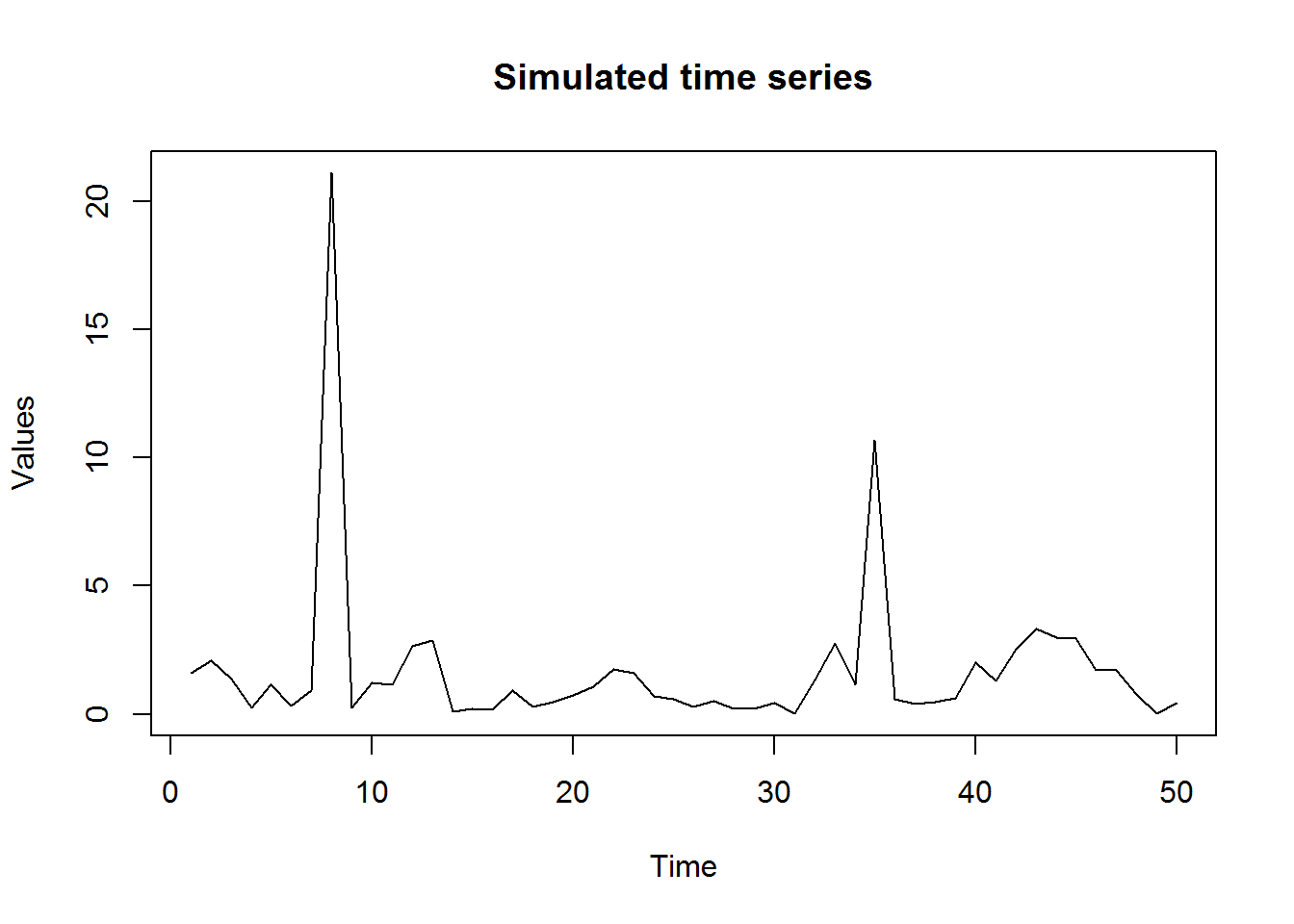## Wednesday 8 April 2015

### The curious case of ARIMA modelling using R

I recently made an interesting observation that I thought is worth sharing. During a data expedition process while trying to fit an ARIMA (auto-regressive moving average) model in order to check for seasonality, I observed something strange while fitting an ARMA model using the function arma{tseries} and arima{stats} in R.

The description of the above 2 procedures is fairly detailed and well explained in the package documentation. On a high level the critical difference between the estimation methods in the two procedures is following:

1. arma{tseries}

arma uses optim to minimize the conditional sum-of-squared errors. The gradient is computed, if it is needed, by a finite-difference approximation. Default initialization is done by fitting a pure high-order AR model (see ar.ols). The estimated residuals are then used for computing a least squares estimator of the full ARMA >model. See Hannan and Rissanen (1982) for details.

1. arima{stats}

The exact likelihood is computed via a state-space representation of the ARIMA process, and the innovations and their variance found by a Kalman filter. The initialization of the differenced ARMA process uses stationarity and is based on Gardner et al (1980).

Above information is cited directly from the package documentation in R.

Given the difference in the estimation procedures between the two functions, one would not expect the results to be radically different for the two function if we use the same timeseries.

Well seems that they can!

Let me illustrate this using an example. First, let us simulate the “interesting” timeseries. (Dont ask me how I generate it, I am not at the discretion to disclose that and also, it is not important for the argument).

set.seed(1010)
ts.sim <- abs(arima.sim(list(order = c(1,0,0), ar = 0.7), n = 50))
ts.sim <- ts.sim*8
ts.sim <- ts.sim*8

Here is how the series looks:Here is the stationarity test results:

library(tseries)
adf.test(ts.sim, k = 0)
##
##  Augmented Dickey-Fuller Test
##
## data:  ts.sim
## Dickey-Fuller = -7.0829, Lag order = 0, p-value = 0.01
## alternative hypothesis: stationary
adf.test(ts.sim, k = 1)
##
##  Augmented Dickey-Fuller Test
##
## data:  ts.sim
## Dickey-Fuller = -4.9149, Lag order = 1, p-value = 0.01
## alternative hypothesis: stationary
adf.test(ts.sim, k = 2)
##
##  Augmented Dickey-Fuller Test
##
## data:  ts.sim
## Dickey-Fuller = -4.0236, Lag order = 2, p-value = 0.01576
## alternative hypothesis: stationary

The series is clearly stationary as indicated by the adf.test. Keep aside the skepticism that the small sample size is biasing the results, also the simulated timeseries is nothing but an arma(1,0) series with AR coefficient as 0.7 and two outlier values induced.

Now lets try fitting an Auto-regressive moving average model to this data using the above two competing approaches and see how the results span out.

arma(ts.sim, order = c(1,0))
##
## Call:
## arma(x = ts.sim, order = c(1, 0))
##
## Coefficient(s):
##       ar1  intercept
##  -0.03963    1.76927
arima(ts.sim, order = c(1,0,0))
##
## Call:
## arima(x = ts.sim, order = c(1, 0, 0))
##
## Coefficients:
##           ar1  intercept
##       -0.0389     1.7002
## s.e.   0.1401     0.4366
##
## sigma^2 estimated as 10.27:  log likelihood = -129.18,  aic = 264.36

Let us make a small tweak to our simulated timeseries by shifting the entire timeseries by a factor of one billion.

summary(ts.sim)
##    Min. 1st Qu.  Median    Mean 3rd Qu.    Max.
##  0.0131  0.4036  0.9162  1.6990  1.7030 21.1200
ts.sim.1 <- ts.sim*1000000000
options(scipen = 999)
summary(ts.sim.1)
##        Min.     1st Qu.      Median        Mean     3rd Qu.        Max.
##    13100000   403600000   916200000  1699000000  1703000000 21120000000

Not let us try and fit the same two models on this revised data that had the level shifted by a factor of a billion.

arma(ts.sim.1, order = c(1,0))
## Warning in arma(ts.sim.1, order = c(1, 0)): Hessian negative-semidefinite
##
## Call:
## arma(x = ts.sim.1, order = c(1, 0))
##
## Coefficient(s):
##              ar1         intercept
##         -0.03959  1769409008.54518

The output above makes sense if you think about it. The coefficient of the AR term remains pretty much the same as what it was in the ARMA model on the original simulated series but the intercept has changed from 1 to 1 Billion to capture the change in the level. There is a warning that the Hessian is negative semi-definite but the estimation happens nevertheless and produces fairly reasonable output.

arima(ts.sim.1, order = c(1,0,0))
## Error in solve.default(res\$hessian * n.used, A): system is computationally singular: reciprocal condition number = 1.90892e-19

Oops. Thats a bummer, din’t expect that to happen!?!

I understand that there is a radical difference in the estimation procedures adopted by the two functions, Kalman filter in arima{stats} as opposed to ML estimation in arma{tseries} but the ability of arima{stats} to not be able to estimate the arma model at all made me a little uncomfortable. Where it gets more tricky is when the output of arima{stats} is a dependency for other packages for eg. x12{x12} that uses the output of arima{stats} as an input.

Where I figured out this problem was when SAS software was successfully able to run the proc x12 procedure to conduct the seasonality test but the same function on R gave me the error above. That made me really wonder and look at the SAS results with skepticism but it turn out, it might just be something to do with the arima{stats}.

Guess it is a curious case after all!